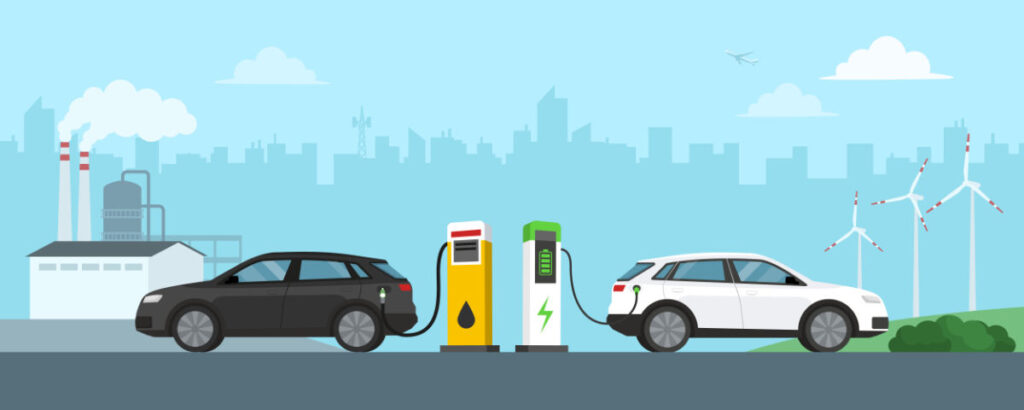# Consumers: Translating Performance## Translating Performance

Many factors go into deciding which car to buy. Colour, design, and safety, are all important considerations, but you will also want to know about the car’s performance. How much power does the car have? How much does it cost to fill the tank, and what sort of fuel efficiency does the vehicle have?

When buying a conventional gasoline-powered vehicle, we use conventional terms to help answer these questions. For instance, asking how much power a vehicle has is usually answered by stating the horsepower (HP) of the engine. Inquiring about the fuel efficiency of a conventional car is answered by explaining the vehicle’s miles-per-gallon, or litres-per-100 km ratings, and/or how many litres the gas tank holds.

But when buying an electric vehicle, we need to ask a different set of questions. For instance – refueling an electric vehicle becomes recharging a vehicle, and the power of an electric motor is expressed in  Kilowatts (kW) rather than horsepower (HP).

Let’s explain:

Horsepower (how fast does it go, how fast can it accelerate) it is the conventional rating for a vehicle’s power. Power for EVs is measured in kilowatts and refers the maximum power handling capacity of the drivetrain.

Horsepower and kilowatts can be converted back and forth.

1. One horsepower is roughly three-quarters of a kilowatt, or 0.75 kW.
2. Multiply HP by ¾ (0.75) to get kW.
3. Multiply kW by 1.34 to get HP.

Nissan’s first generation LEAF was equipped with an 80kW A/C motor. 80 x 1.34=107.2HP. The LEAF ePlus is equipped with a 160kW motor, equivalent to 215HP

Capacity is typically measured in litres-per -100 km for a conventional gas-powered vehicle. In electric vehicles, capacity is measured in kilowatt hours, or kWh. When we think of capacity, we think of how long we can drive the car. For EVs, instead of litres of gas consumed, we think in terms of kilowatt hours.

Most EVs are equipped with a trip computer, allowing the driver easy access to energy efficiency calculations. Vehicles normally measure consumption in watt-hours per mile or watt-hours per km. Basically, if we have a 100kWh battery and an efficiency of 5kms per kWh, then 100kWh times 5km per kWh = 500km per-charge range. Parasitic losses from computers, heating and cooling, and friction can guzzle a little extra power, but regenerative braking can harvest and recover almost 40% of spent energy in low speed city driving.